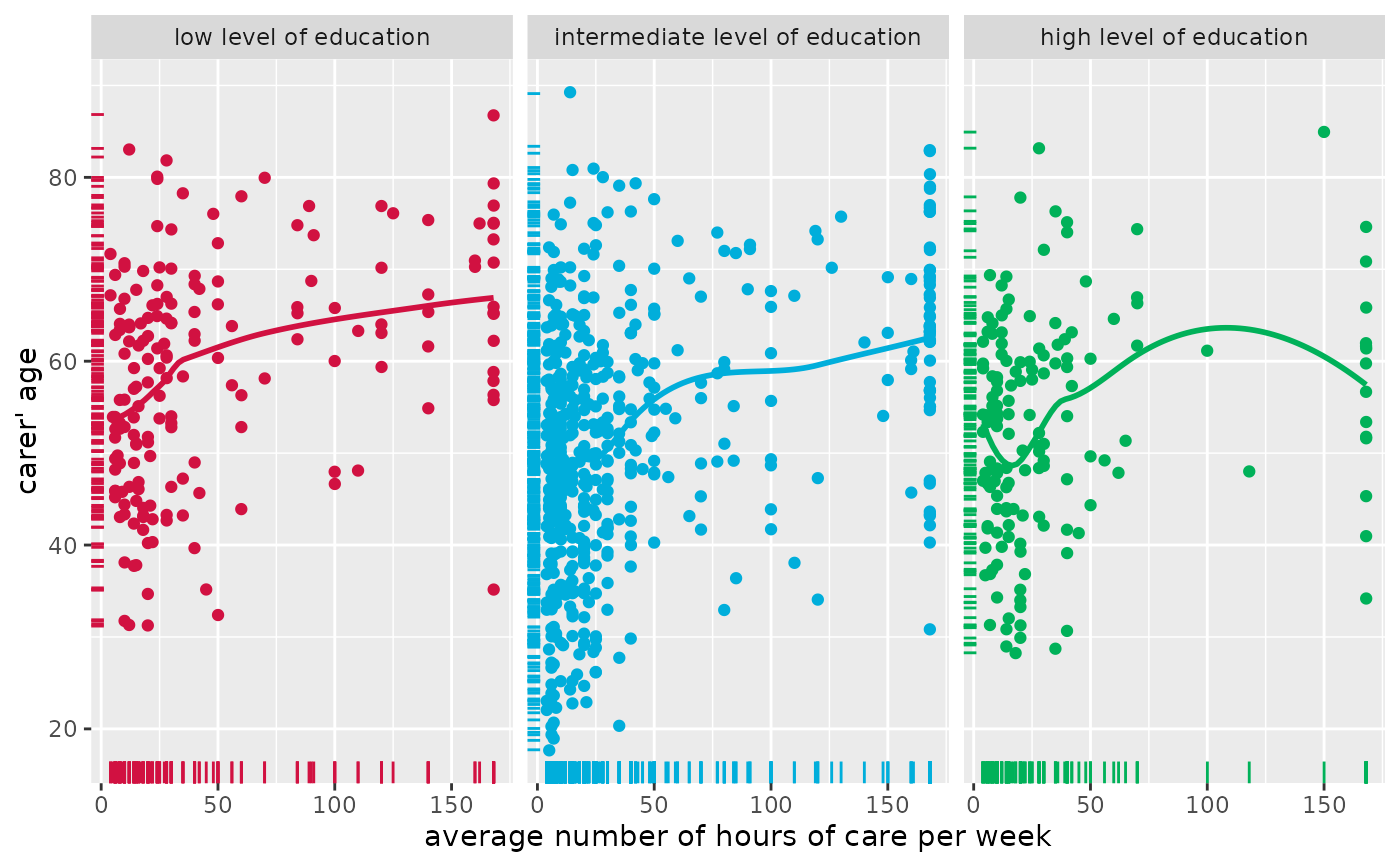Display scatter plot of two variables. Adding a grouping variable to the scatter plot is possible. Furthermore, fitted lines can be added for each group as well as for the overall plot.

## Usage

plot_scatter(
data,
x,
y,
grp,
title = "",
legend.title = NULL,
legend.labels = NULL,
dot.labels = NULL,
axis.titles = NULL,
dot.size = 1.5,
label.size = 3,
colors = "metro",
fit.line = NULL,
fit.grps = NULL,
show.rug = FALSE,
show.legend = TRUE,
show.ci = FALSE,
wrap.title = 50,
wrap.legend.title = 20,
wrap.legend.labels = 20,
jitter = 0.05,
emph.dots = FALSE,
grid = FALSE
)

## Arguments

data

A data frame, or a grouped data frame.

x

Name of the variable for the x-axis.

y

Name of the variable for the y-axis.

grp

Optional, name of the grouping-variable. If not missing, the scatter plot will be grouped. See 'Examples'.

title

Character vector, used as plot title. By default, response_labels is called to retrieve the label of the dependent variable, which will be used as title. Use title = "" to remove title.

legend.title

Character vector, used as legend title for plots that have a legend.

legend.labels

character vector with labels for the guide/legend.

dot.labels

Character vector with names for each coordinate pair given by x and y, so text labels are added to the plot. Must be of same length as x and y. If dot.labels has a different length, data points will be trimmed to match dot.labels. If dot.labels = NULL (default), no labels are printed.

axis.titles

character vector of length one or two, defining the title(s) for the x-axis and y-axis.

dot.size

Numeric, size of the dots that indicate the point estimates.

label.size

Size of text labels if argument dot.labels is used.

colors

May be a character vector of color values in hex-format, valid color value names (see demo("colors")) or a name of a pre-defined color palette. Following options are valid for the colors argument:

• If not specified, a default color brewer palette will be used, which is suitable for the plot style.

• If "gs", a greyscale will be used.

• If "bw", and plot-type is a line-plot, the plot is black/white and uses different line types to distinguish groups (see this package-vignette).

• If colors is any valid color brewer palette name, the related palette will be used. Use RColorBrewer::display.brewer.all() to view all available palette names.

• There are some pre-defined color palettes in this package, see sjPlot-themes for details.

• Else specify own color values or names as vector (e.g. colors = "#00ff00" or colors = c("firebrick", "blue")).

fit.line, fit.grps

Specifies the method to add a fitted line accross the data points. Possible values are for instance "lm", "glm", "loess" or "auto". If NULL, no line is plotted. fit.line adds a fitted line for the complete data, while fit.grps adds a fitted line for each subgroup of grp.

show.rug

Logical, if TRUE, a marginal rug plot is displayed in the graph.

show.legend

For Marginal Effects plots, shows or hides the legend.

show.ci

Logical, if TRUE), adds notches to the box plot, which are used to compare groups; if the notches of two boxes do not overlap, medians are considered to be significantly different.

wrap.title

Numeric, determines how many chars of the plot title are displayed in one line and when a line break is inserted.

wrap.legend.title

numeric, determines how many chars of the legend's title are displayed in one line and when a line break is inserted.

wrap.legend.labels

numeric, determines how many chars of the legend labels are displayed in one line and when a line break is inserted.

jitter

Numeric, between 0 and 1. If show.data = TRUE, you can add a small amount of random variation to the location of each data point. jitter then indicates the width, i.e. how much of a bin's width will be occupied by the jittered values.

emph.dots

Logical, if TRUE, overlapping points at same coordinates will be becomme larger, so point size indicates amount of overlapping.

grid

Logical, if TRUE, multiple plots are plotted as grid layout.

## Value

A ggplot-object. For grouped data frames, a list of ggplot-objects for each group in the data.

## Examples

# load sample date
library(sjmisc)
library(sjlabelled)
data(efc)

# simple scatter plot
plot_scatter(efc, e16sex, neg_c_7)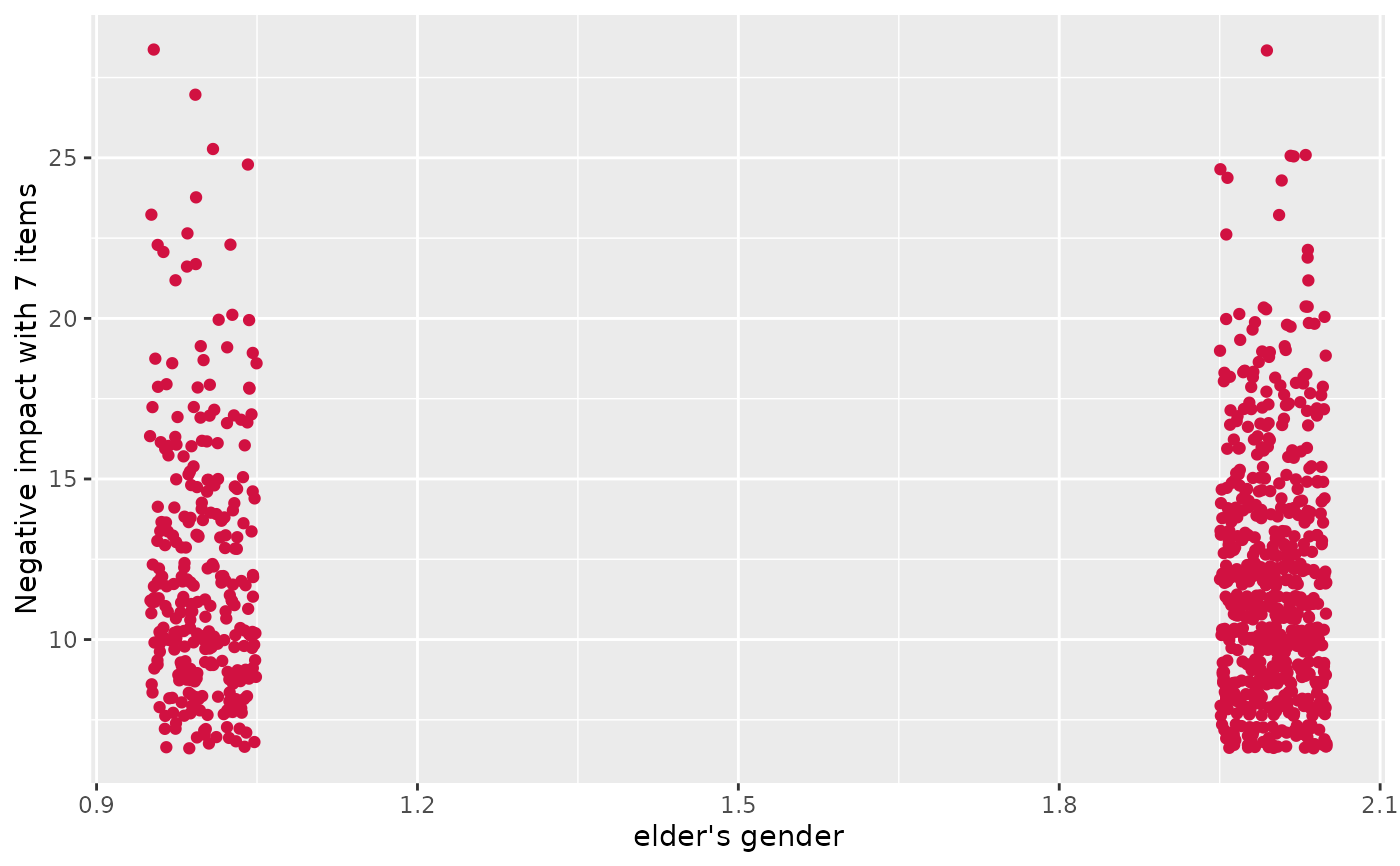# simple scatter plot, increased jittering
plot_scatter(efc, e16sex, neg_c_7, jitter = .4)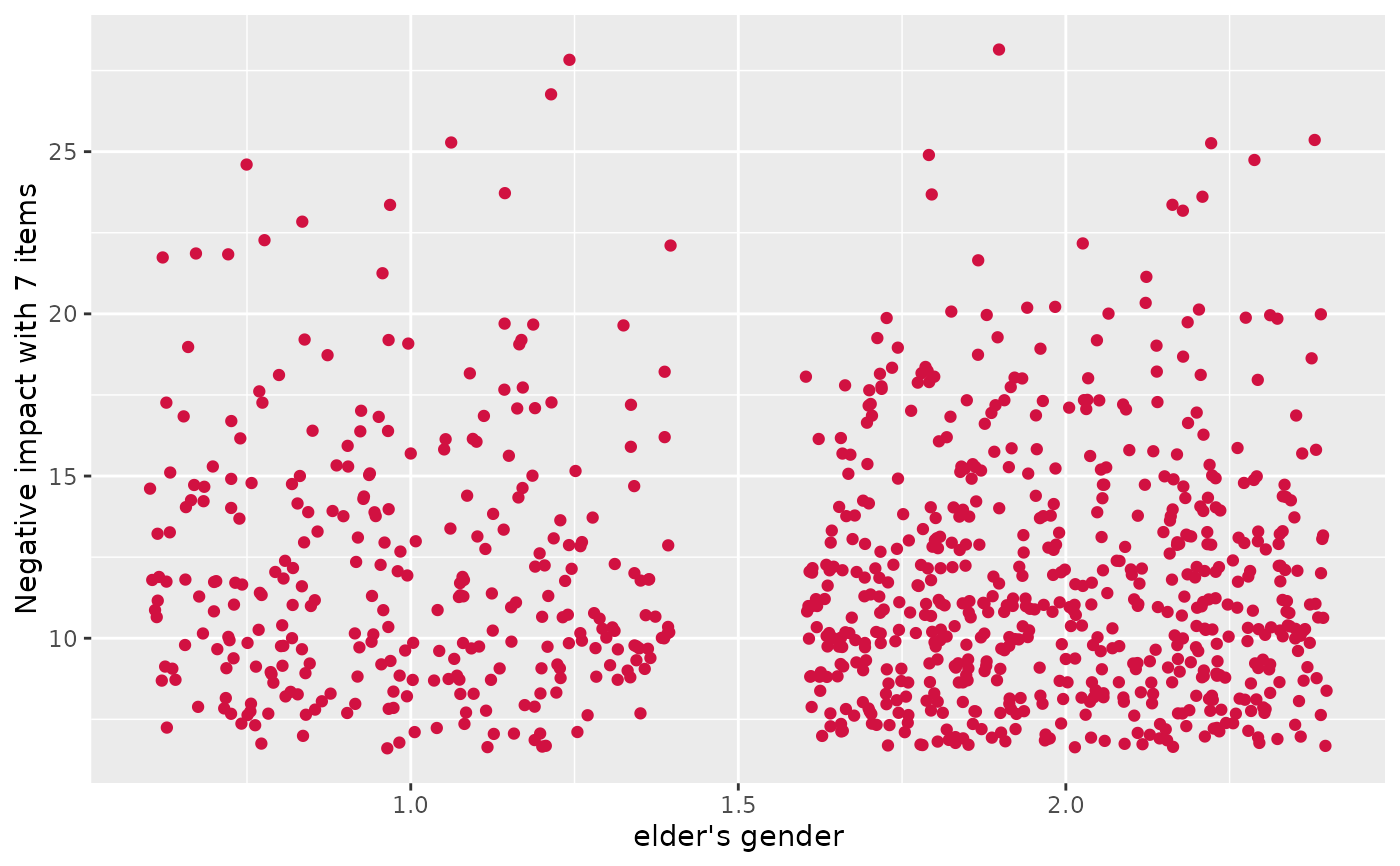# grouped scatter plot
plot_scatter(efc, c160age, e17age, e42dep)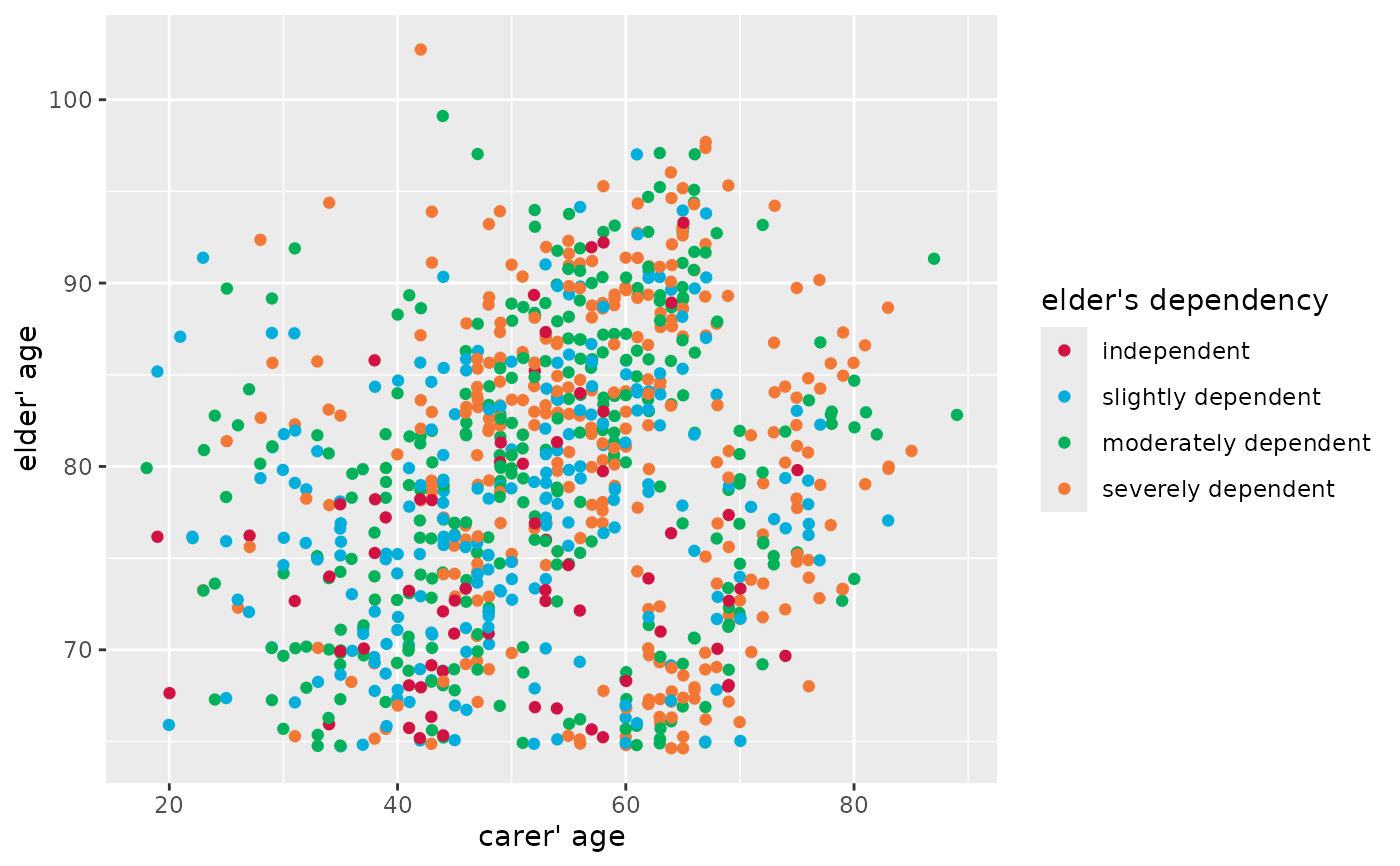# grouped scatter plot with marginal rug plot
# and add fitted line for complete data
plot_scatter(
efc, c12hour, c160age, c172code,
show.rug = TRUE, fit.line = "lm"
)
#> geom_smooth() using formula = 'y ~ x'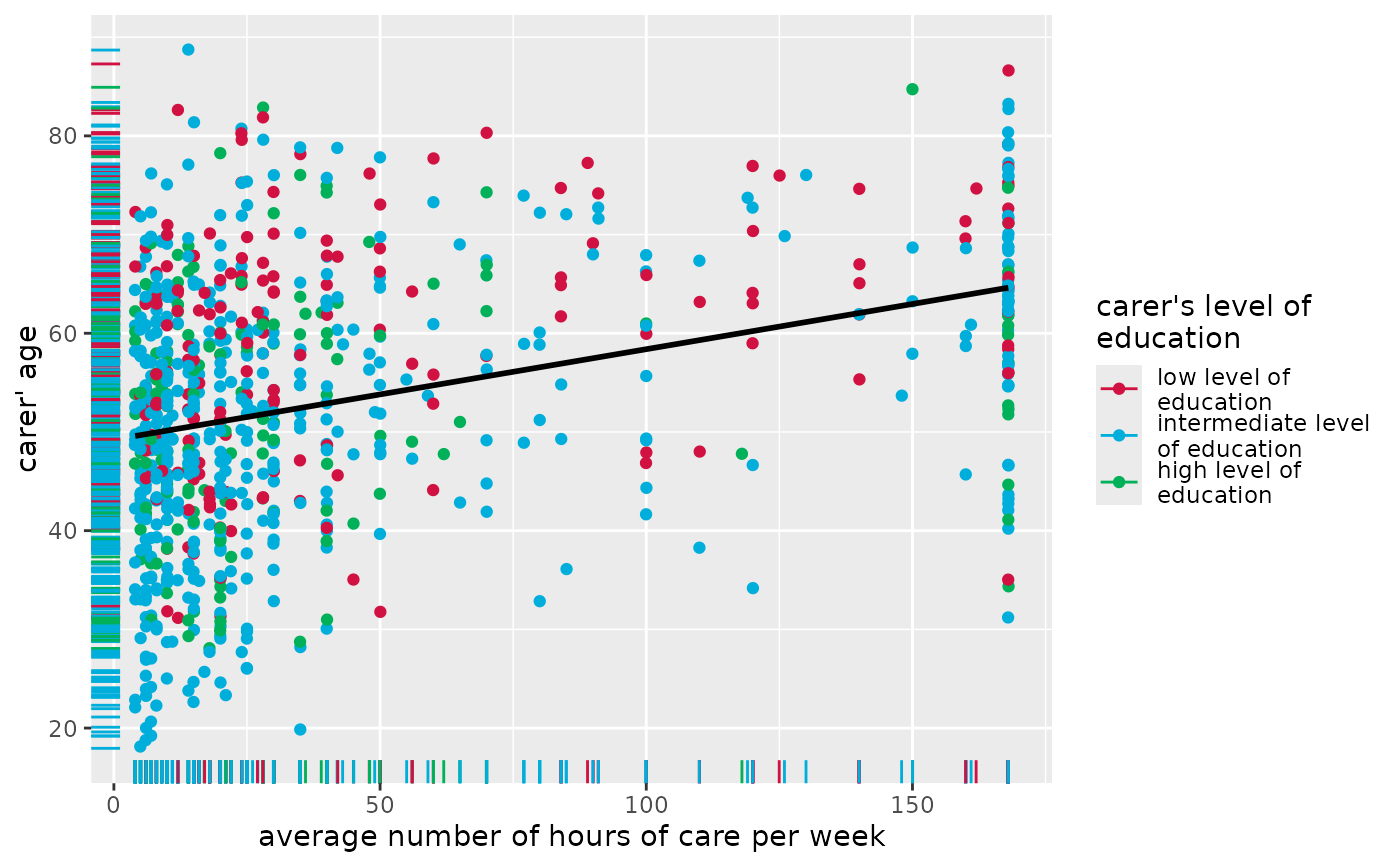# grouped scatter plot with marginal rug plot
# and add fitted line for each group
plot_scatter(
efc, c12hour, c160age, c172code,
show.rug = TRUE, fit.grps = "loess",
grid = TRUE
)
#> geom_smooth() using formula = 'y ~ x'